# Algebra 1 : Graphing Inequalities

## Example Questions

2 Next →

### Example Question #11 : Graphing Inequalities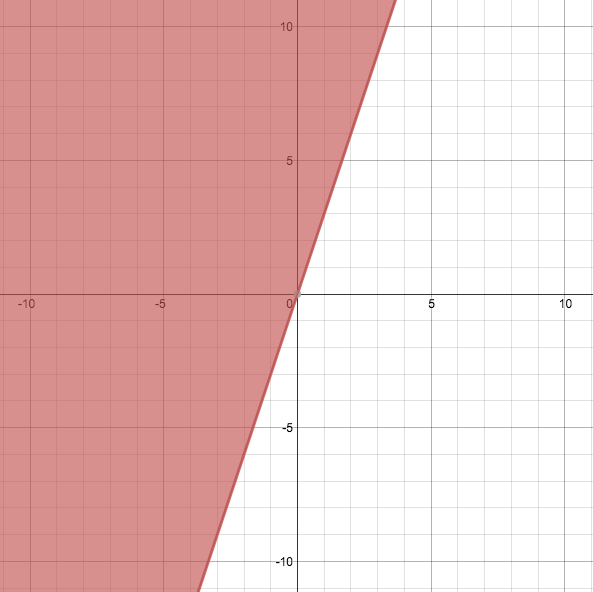The above graph depicts which of the following equations or inequalities?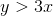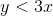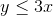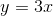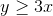Explanation:

Given the above graph, we can initially deduce that, andare not the correct answer. The dashed line in the graph indicates that no point on the lineis a solution to the inequality, and the shaded area indicates that the correct answer must account for points in a certain region beyond. Thus, we're left withand.

We can use a test point to determine which of the remaining inequalities is the correct answer. The test point can be any point that is not on the line, so let's select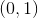in this case. Pluggingintoyields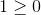. Since this is true, we know that every point on the same side of the line aswill yield a true result, and that our graph represents.

### Example Question #72 : Linear Inequalities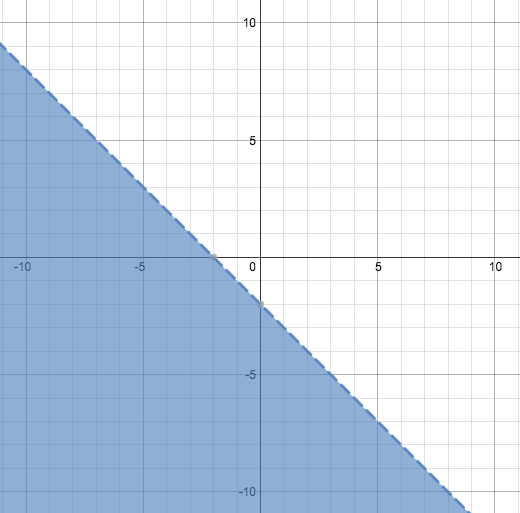The above graph depicts which of the following equations or inequalities?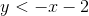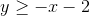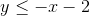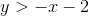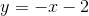Explanation:

Given the above graph, we can initially deduce that,, andare not the correct answer. The dashed line in the graph indicates that no point on the lineis a solution to the inequality, and the shaded area indicates that the correct answer must account for points in a certain region beyond. Thus, we're left withand.

We can use a test point to determine which of the remaining inequalities is the correct answer. The test point can be any point that is not on the line, so let's select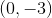in this case. Pluggingintoyields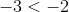. Since this is true, we know that every point on the same side of the line aswill yield a true result, and that our graph represents.

### Example Question #71 : Linear Inequalities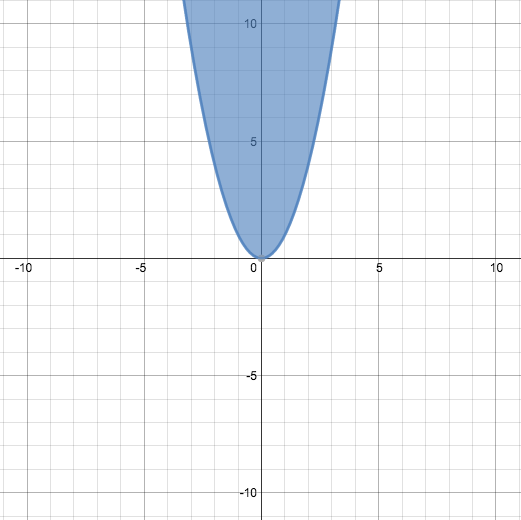The above graph depicts which of the following equations or inequalities?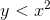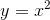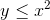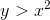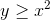Explanation:

Given the above graph, we can initially deduce that, andare not the correct answer. The solid line in the graph indicates that all points on the lineare solutions to the inequality, and the shaded area indicates that the correct answer must account for points in a certain region beyond. Thus, we're left withand.

We can use a test point to determine which of the remaining inequalities is the correct answer. The test point can be any point that is not on the line, so let's select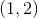in this case. Pluggingintoyields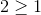. Since this is true, we know that every point on the same side of the line aswill yield a true result, and that our graph represents.

### Example Question #11 : Graphing Inequalities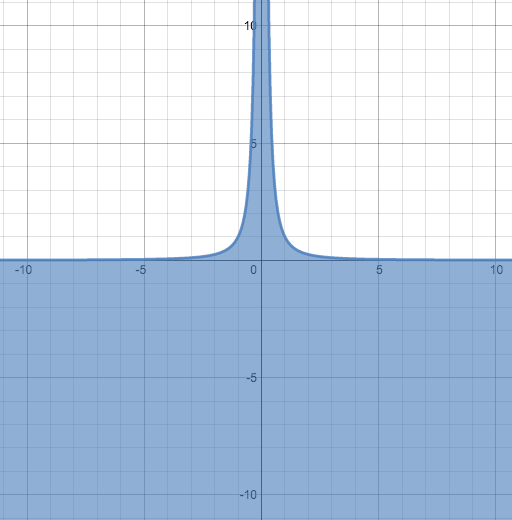The above graph depicts which of the following equations or inequalities?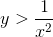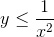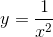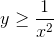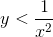Explanation:

Given the above graph, we can initially deduce that, andare not the correct answer. The solid line in the graph indicates that all points on the lineare solutions to the inequality, and the shaded area indicates that the correct answer must account for points in a certain region beyond. Thus, we're left withand.

We can use a test point to determine which of the remaining inequalities is the correct answer. The test point can be any point that is not on the line, so let's select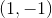in this case. Pluggingintoyields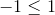. Since this is true, we know that every point on the same side of the line aswill yield a true result, and that our graph represents.

### Example Question #12 : Graphing Inequalities

What is the equation of the graph of the inequality shown below?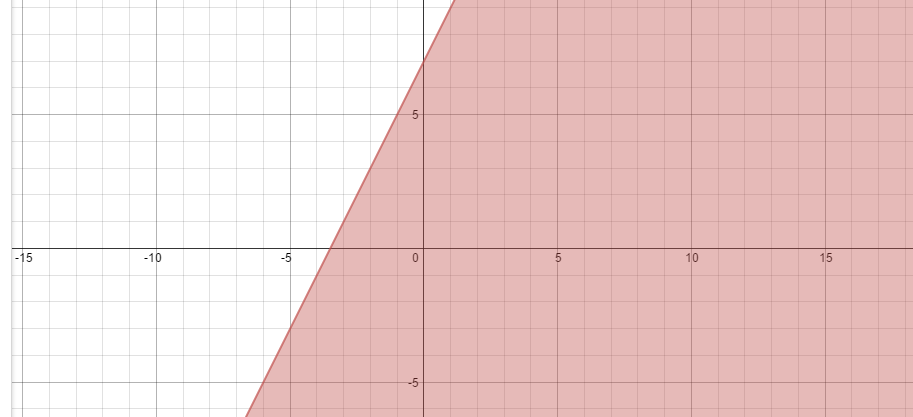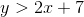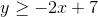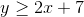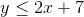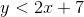Explanation:

Because the line is a solid line and is shaded down you know that the equation is less than or equal to some function.

When finding slope, you must find the rise over the run which is 2. The function also has a y-intercept of 7,### Example Question #76 : Linear Inequalities

Which of the following graphs correctly depicts the graph of the inequalityExplanation:

Let's start by looking at the given equation: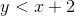The inequality is written in slope-intercept form; therefore, the slope is equal toand the y-intercept is equal to.

All of the graphs depict a line with slope ofand y-intercept. Next, we need to decide if we should shade above or below the line. To do this, we can determine if the statement is true using the origin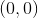. If the origin satisfies the inequality, we will know to shade below the line. Substitute the values into the given equation and solve.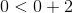Because this statement is true, the origin must be included in the shaded region, so we shade below the line.

Finally, a statement that is "less than" or "greater than" requires a dashed line in the graph. On the other hand, those that are "greater than or equal to" or "less than or equal to" require a solid line. We will select the graph with shading below a dashed line.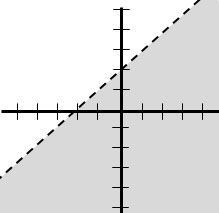2 Next →